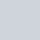Latest MCQs
Home > MCQs > Math > The average age of A and B is 20. If C were to replace A the average would be 19 and if B was replaced by C the average would be 21. The age of C is:

# The average age of A and B is 20. If C were to replace A the average would be 19 and if B was replaced by C the average would be 21. The age of C is:

A.18
B.19
C.20
D.21